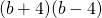Chapter 6: Polynomials

# 6.6 Special Products

There are a few shortcuts available when multiplying polynomials. When recognized, they help arrive at the solution much quicker.

The first is called a difference of squares. A difference of squares is easily recognized because the numbers and variables in its two factors are exactly the same, but the sign in each factor is different (one plus sign, one minus sign). To illustrate this, consider the following example.

Example 6.6.1

Multiply the following pair of binomials: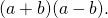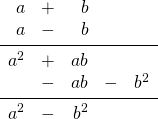Notice the middle term cancels out and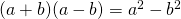. Cancelling the middle term during multiplication is the same for any difference of squares.

Example 6.6.2

Multiply the following pair of binomials: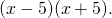Recognize a difference of squares. The solution is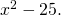Example 6.6.3

Multiply the following pair of binomials: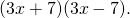Recognize a difference of squares. The solution is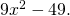Example 6.6.4

Multiply the following pair of binomials: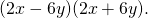Recognize a difference of squares. The solution is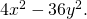Another pair of binomial multiplications useful to know are perfect squares. These have the form of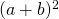or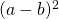.

Example 6.6.5

Multiply the following pair of binomials:and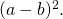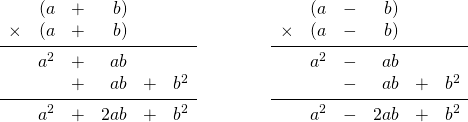The pattern of multiplication for any perfect square is the same. The first term in the answer is the square of the first term in the problem. The middle term is 2 times the first term times the second term. The last term is the square of the last term.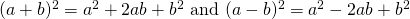Example 6.6.6

Multiply out the following expression: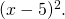Recognize a perfect square. Square the first term, subtract twice the product of the first and last terms, and square the last term.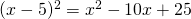Example 6.6.7

Multiply out the following expression: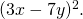Recognize a perfect square. Square the first term, subtract twice the product of the first and last terms, and square the last term.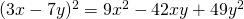Example 6.6.8

Multiply out the following expression: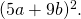Recognize a perfect square. Square the first term, add twice the product of the first and last terms, and square the last term.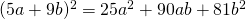# Questions

Find each product.

1.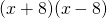2.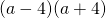3.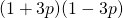4.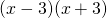5.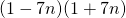6.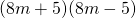7.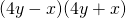8.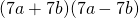9.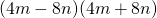10.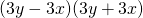11.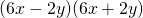12.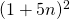13.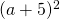14.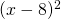15.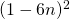16.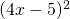17.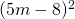18.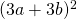19.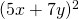20.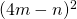21.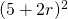22.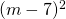23.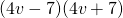24.